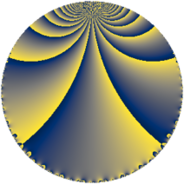Properties

 Label 64.3.dLevel $64$ Weight $3$ Character orbit 64.d Rep. character $\chi_{64}(31,\cdot)$ Character field $\Q$ Dimension $4$ Newform subspaces $1$ Sturm bound $24$ Trace bound $0$

Related objects

Defining parameters

 Level: $$N$$ $$=$$ $$64 = 2^{6}$$ Weight: $$k$$ $$=$$ $$3$$ Character orbit: $$[\chi]$$ $$=$$ 64.d (of order $$2$$ and degree $$1$$) Character conductor: $$\operatorname{cond}(\chi)$$ $$=$$ $$8$$ Character field: $$\Q$$ Newform subspaces: $$1$$ Sturm bound: $$24$$ Trace bound: $$0$$

Dimensions

The following table gives the dimensions of various subspaces of $$M_{3}(64, [\chi])$$.

Total New Old
Modular forms 22 4 18
Cusp forms 10 4 6
Eisenstein series 12 0 12

Trace form

 $$4 q + 12 q^{9} + O(q^{10})$$ $$4 q + 12 q^{9} - 24 q^{17} - 92 q^{25} + 48 q^{33} + 264 q^{41} - 60 q^{49} - 336 q^{57} + 192 q^{65} + 232 q^{73} - 396 q^{81} - 408 q^{89} + 104 q^{97} + O(q^{100})$$

Decomposition of $$S_{3}^{\mathrm{new}}(64, [\chi])$$ into newform subspaces

Label Dim. $$A$$ Field CM Traces $q$-expansion
$a_{2}$ $a_{3}$ $a_{5}$ $a_{7}$
64.3.d.a $4$ $1.744$ $$\Q(\zeta_{12})$$ None $$0$$ $$0$$ $$0$$ $$0$$ $$q-\zeta_{12}q^{3}-\zeta_{12}^{2}q^{5}-\zeta_{12}^{3}q^{7}+3q^{9}+\cdots$$

Decomposition of $$S_{3}^{\mathrm{old}}(64, [\chi])$$ into lower level spaces

$$S_{3}^{\mathrm{old}}(64, [\chi]) \cong$$ $$S_{3}^{\mathrm{new}}(8, [\chi])$$$$^{\oplus 4}$$$$\oplus$$$$S_{3}^{\mathrm{new}}(32, [\chi])$$$$^{\oplus 2}$$